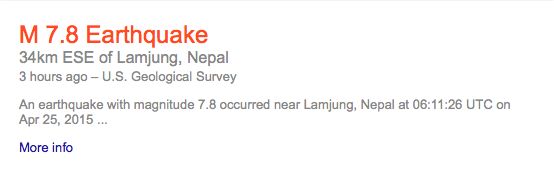## Saturday, April 25, 2015

### 44 78 | Lamjung, Nepal Earthquake of April 25, 2014 (7.8 Magnitude)Notice the time of the Earthquake, 6:11:26 UTC

Today is ripe for an earthquake, why?
• Earthquake = 5+1+9+2+8+8+3+1+2+5 = 44
• 4/25/15 = 4+25+15 = 44
• Kill = 11+9+12+12 = 44
Notice that it was a 7.8 Magnitude Quake, near 'Lamjung'.
• Lamjung = 12+1+13+10+21+14+7 = 78
• Nepal = 14+5+16+1+12 = 48
• Lamjung, Nepal = 126
Today is the 115th day of the year, a day ripe for killing beyond its '44 numerology'.

#### 1 comment:

1.26.5333 + 86.7333 = 113.2666
26.5333 - 86.7333 = -60.2